# Hybridization

Hybridization is the idea that atomic orbitals fuse to form newly hybridized orbitals, which in turn, influences molecular geometry and bonding properties. Hybridization is also an expansion of the valence bond theory. In order to explore this idea further, we will utilize three types of hydrocarbon compounds to illustrate sp3, sp2, and sp hybridization.

## Methane

First and foremost, it is important to note that carbon has the electron configuration of 1s2 2s2 2p2. This means that carbon would have 2 unpaired electrons in its p orbitals. Theoretically, this means that carbon will only form 2 bonds, but that is definitely not the case.As seen in methane (CH4), carbon can form 4 bonds. The rationale behind this phenomenon is hybridization. Supporting evidence shows that 1 s and 3 p orbitals are being combined to form hybrid orbitals, allowing polyatomic molecule to have 25% s character and 75% p character. Thus, we call methane a sp3-hybridized molecule.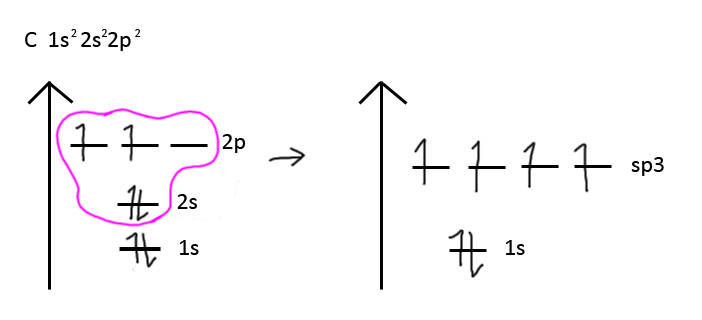The above figure also explains why the four C-H bonds are equivalent to one another. Initially, people thought that if methane had four C-H bonds, that would mean there would be three of one particular type, and one of another type. Hence, this is also something that hybridization explains. Nonetheless, there is even mathematical proof to support this concept! These equations together not only break down what the composition of each hybridized orbital, but also portray the possible directions each orbital can orient in a 3D plane.Don’t let the equations scare you. Carefully witness pictorally how each individual hybridized orbital is comprised of the 25% s character and 75% p character. Then witness how the combined orbitals form the sp3 tetrahedral shape.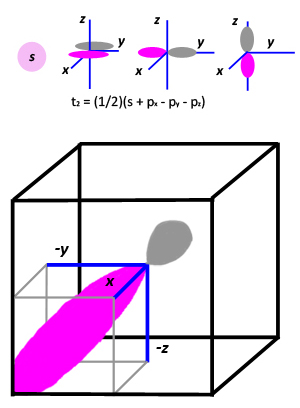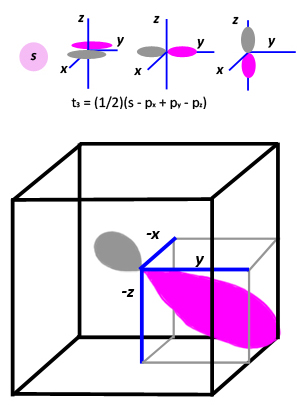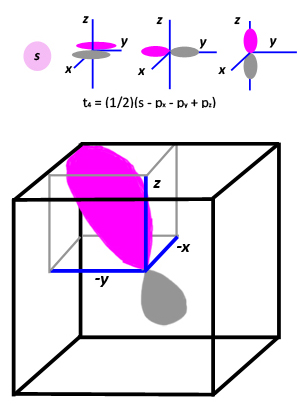The take home message is to note that in the end, you’ll have 4 hybridized orbitals orienting as far away from each other as possible. Thus, hybridization explains why there is a 109 degree angle between orbitals.## Ethylene

Unlike methane, ethylene is shaped differently, despite the fact that the carbon in ethylene has the same electron configuration. What accounts for this difference? Supporting evidence shows that the carbon in an ethylene molecule is sp2 hybridized. This means that 1 s orbital is being mixed with 2 p orbitals.This time, the energy diagram setup is different because only 2 p orbitals are being mixed. While creating your energy diagram, however, be sure that you do not make the mistake as shown above. By placing two electrons in the same orbital, you have broken Hund's rule, which states that all orbitals among the same energy levels have to be filled with at least one electron before being paired up again. The 2p orbital here is considered low enough energy to be classified within the same energy level as the sp2 orbitals. The figure below portrays the correct way to distribute your electrons.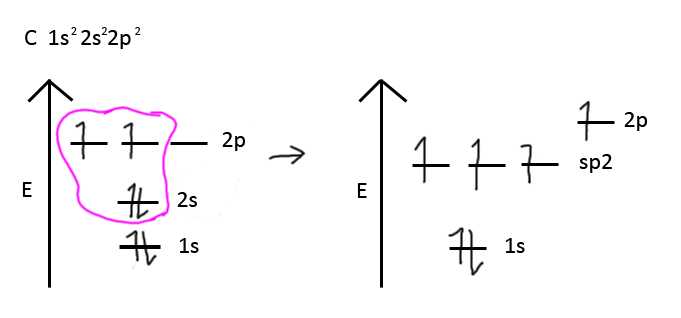Notice how that lone electron in the 2p orbital is separate from the electrons in the sp2 orbitals. This is what influence ethylene's shape. The lone electron from each carbon will remain in its respective p orbital and form a pi bond with the other p orbital electron. Thus, ethylene is a planar molecule, with orbitals spaced 120 degree angles apart.In case you can't visualize this picture in 3D, allow me to clarify: the hot pink sp2 hybridized orbitals are all on the same plane (let's say among the xy plane), whereas the pi bonds are running along in a plane perpendicular to the xy plane.

Nonetheless, the figure above accurately depicts the ethylene Lewis structure: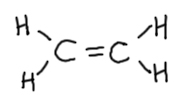The double bond in the lewis structure is indicative of one sigma bond and one pi bond.

## Acetylene

Supporting evidence shows that acetylene is an sp molecule. This means that 1 s orbital is being mixed with 1 p orbital. Thus, the energy diagram setup would look like this:As previously discussed, the lone electrons in the 2p orbitals are not part of the sp orbitals. Instead, each electron is in its respective p orbital, and will bond with its respective p orbital of the other carbon. This in itself will create a sigma bond and two pi bonds, leading to the formation of a linear molecule!Thus, in a lewis structure, acetylene is comprised of 2 triple-bond carbons:As you can see, the bond angle is 180 degrees, indicative of a linear molecule. This information is consistent with what was explained earlier.

## References

1. Chang, Raymond. "Hybridization of Atomic Orbitals." Physical Chemistry for the Biosciences. Sansalito, CA: University Science, 2005. 450-55. Print.
2. Petrucci, Ralph H. "Hybridization of Atomic Orbitals." General Chemistry: Principles and Modern Applications. 9th ed. Upper Saddle River, NJ: Pearson/Prentice Hall, 2007. Print.